Using JMP > Enter and Edit Your Data > Restructure Data > Make a Binning Formula in a Data Table Column
Publication date: 04/12/2021

## Make a Binning Formula in a Data Table Column

You can distribute your data into bins using the Make Binning Formula option. Select the column or columns that you want to divide into bins, and select Cols > Utilities > Make Binning Formula. A histogram of the bins and a Value Label legend are shown, along with a red triangle menu that contains options for defining the cutpoints. There are several ways to add cutpoints, remove cutpoints, and adjust the bin sizes.

Right-click in the histogram to add or remove cutpoints.

Click and drag the vertical lines in the histogram to adjust the bin sizes.

Edit the boxes next to the cutpoint value labels to edit the cutpoint values and adjust the bin sizes.

Use the add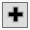and remove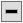buttons above the Value Label legend to add and remove rows, which is equivalent to adding and removing cutpoints.

Use the undo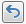and redo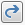buttons to undo or redo actions done on the bin settings.

There is an option to add formula columns for the cutpoints to the data table.

### Right-Click Options for Make Binning Formula

Right-click the histogram in the Make Binning Formula window to select the following options:

Add Cutpoint

Adds a cutpoint line to the histogram in the location that was clicked.

Remove Cutpoint

Removes a cutpoint line. This option is available only if you right-click on an existing cutpoint.

Show Counts

Shows or hides the counts above the bars.

Show Percents

Shows or hides the percentages above the bars.

Subset

Creates a subset data table based on the current selection.

Fill Pattern

Specifies the fill pattern for the bars in the histogram.

### Red Triangle Options for Cutpoints

The Cutpoints red triangle contains the following options:

Fill using Equal Width Bins

Specifies a start (offset) and a bin width. JMP fills in the table until it goes past the largest possible values. For example, if you enter offset=50 and width=10 and your largest data value is 95, you will get the following bins: 50, 60, 70, 80, 90, 100.

Fill using Bin Count

Specifies a start, end, and a bin count. JMP divides the space between the start and end equally by the bin count. If you change the bin count, the reported bin width is automatically updated in the window.

Fill using Percentiles

Specifies a percentage between 1 and 50. JMP divides the range of the data by that quantile. For example, if you specify a percentile of 20, you get five equal weight bins.

Fill using Mean/StdDev

Specifies the bin cutpoints to be set at the mean of the data plus the first, second, or third standard deviation from the mean based on your selection in the window.

Fill using Jenks Natural Breaks

Specifies a bin count and creates bin cutpoints so that the variance within each bin is minimized and the variance between the bins is maximized.

Bin Label

Specifies whether value labels are shown instead of the data values.

Use Value Labels

Shows a label instead of the value shown in the new formula column defining the bins.

Use Range Labels

Includes the lower and upper values for each range in the label.

No Labels

Uses the lower edge value as the label.

Character

Applies the Character data type. The formula produces the labels.

See Value Labels.

Tip: Value Labels are recommended in most platforms, many of which do not support range labels. In the Categorical platform, you must use value labels. On some axes, you might find that range labels more clearly identify the values, or you can try adjusting the offset and width for the axis.

Bin Label Style

Specifies a preset that defines what the Value Labels or Character output look like in the table.

LowHigh

Defines the Value Labels by the lowest and highest points of each bin. The format is LowHigh.

Low to High

Defines the Value Labels by the lowest and highest points of each bin. The format is Low to High.

Low, High

Defines the Value Labels by the lowest and highest points of each bin. The format is Low, High.

LowHigh-1 (integers)

Defines the Value Labels by the lowest point and one less than the highest point of each bin. The format is LowHigh-1.

Low to High-1 (integers)

Defines the Value Labels by the lowest point and one less than the highest point of each bin. The format is Low to High-1.

[Low, High) (mathematical)

Specifies that the lower value is included in the range and the upper value is not. For example, if your range is [50,60), then a value of 50 would be in the bin, but a value of 60 would not be.

Low

Defines the Value Labels by the lowest point of each bin.

Midpoint

Defines the Value Labels by the midpoint of each bin.

Integer Sequence

Defines the Value Labels by a sequence of consecutive integers. Define the starting integer in the Integer Sequence Labels window.

Uppercase Alphabetical

Defines the Value Labels as uppercase letters in alphabetical order.

Lowercase Alphabetical

Defines the Value Labels as lowercase letters in alphabetical order.

Custom

Enables you to enter custom Value Labels.

Column Format

Specifies how the Value Labels are formatted. By default, the format of the column is applied. However, you might want to override the setting (for example, changing the format to Dollar). This setting is most useful if the column format is something like Best, but you really want your bins to have no decimals. Instead of cleaning up all of the value labels, you specify the format.

Histogram

Specifies whether counts or percentages appear above the bars.

Show Points

Shows or hides the observed data points below the bars.

Rescale Axis

Resets the axis to the original scale.

Recall

Populates the Make Binning Formula window with the last binning actions that you performed.

Make All Like X

(Appears only if multiple columns are selected.) Applies the choices made for the first column (X) to the remaining columns in the dialog.

Make Formula Columns

Creates the formula columns and closes the window.

Tip: Once you create a formula column, you can edit the formula by right-clicking on the column header and selecting Edit Binning Formula. This reopens the Make Binning Formula window. To update the binning formula column, edit the cutpoints and click Update Column.

#### Example of Making a Binning Formula

1. Select Help > Sample Data Library and open Big Class.jmp.

2. Select the height column.

3. Select Cols > Utilities > Make Binning Formula.

You want the range of values to appear as X-X, so in the Bin Label Style, keep the range set to Low - High.

4. Click the Cutpoints red triangle and select Fill using Equal Width Bins.

5. In the window that appears, change the offset to -0.5.

Tip: For integer data, setting the offset to -0.5 helps disambiguate values on the edge. In this example, one of the bins covers 59.5 to 64.5, so it is clear that 59 and 65 are not included in this bin.

6. Keep the width set to 5.

7. Click OK.

8. Keep the Bin Label setting of Use Value Labels so that you can see the range of values for the bin.

Figure 4.39 Completed Binning Window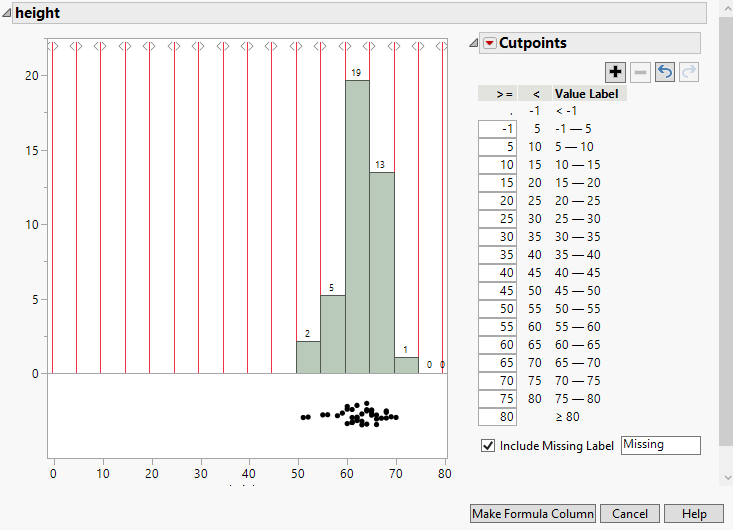9. Click Make Formula Column.

A column called height Binned is added to the Big Class.jmp data table.

10. To see how the formula is calculated, right-click the height Binned column and select Formula.

Figure 4.40 Formula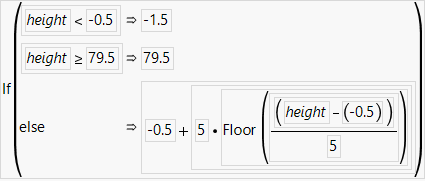Want more information? Have questions? Get answers in the JMP User Community (community.jmp.com).